Aerial Map Calculator
Area Totals
Current Area
0 ft2
Total Area
0 ft2
Total Measurement: ft2
Total Price: \$0
Square Footage Calculator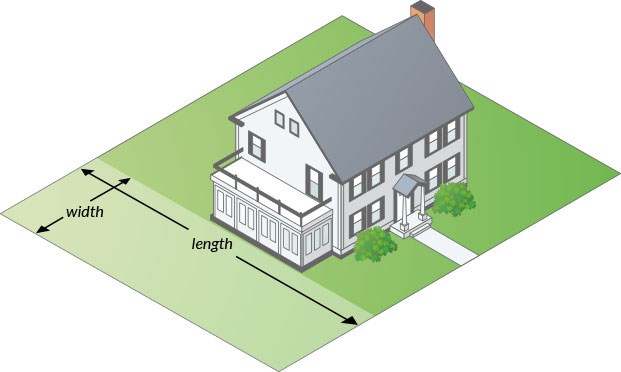#### How to measure your lawn for sod.

When purchasing sod, it’s important to have enough to provide full coverage. Measure the length and width of each area you plan to sod and enter the totals below to calculate the exact amount you need to order for your project.

Sod Roll: 6.5 ft x 2 ft = 13 ft2
Sod Pallet: 54 rolls per pallet = 702 ft2

• Area 1
• Area 1
• Area 1
Total Measurement: 0 ft2
Total Price: \$0

### How To Measure

#### Square or Rectangle

Measure the Length & Width and multiply.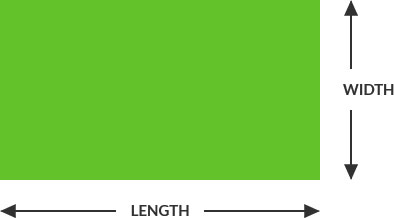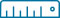Length x Width = Area
(12’ x 24’ = 288 ft2)

#### Right Triangle

Multiply the two sides that contact the right angle and divide by two.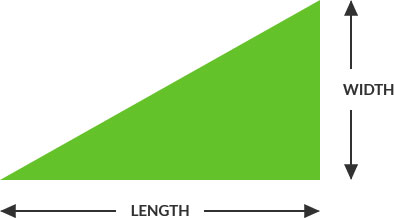Length x Width / 2 = Area
(12’ x 24’ = 288 ÷ 2 = 144 ft2)

#### Circular

Multiply the radius with the radius then multiply by 3.14. Radius is a straight line from the center of a circle to the outside of the circle.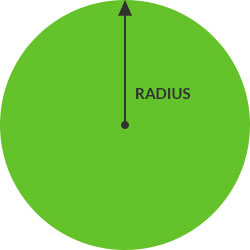##### Full Circle:(6’ x 6’ = 36 x 3.14 = 113 ft2)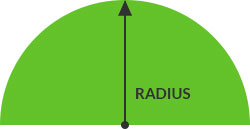##### Half Circle:Divide full circle radius by 2
(6’ x 6’ = 36 x 3.14 = 113 / 2 = 56.5 ft2)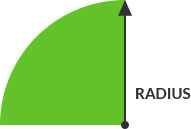##### Quarter Circle:Divide full circle radius by 4
(6’ x 6’ = 36 x 3.14 = 113 / 4 = 28.25 ft2)